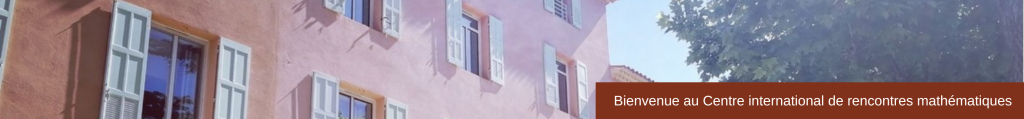RESEARCH IN PAIRS

Properties of Edit Distances between Signed and Unsigned Permutations
July, 6 – 17, 2015
 We study edit distances between permutations of n elements, defined as the minimum number of operations (from some given set of allowed transformations, fixed in advance) needed to transform a given permutation into the identity permutation (1 2 … n). Some well-studied examples of such transformations include reversals, which reverse the order of elements in a given interval; element exchanges, which swap any two elements of the permutation; and block-transpositions, which swap adjacent, non-intersecting intervals in the permutation. We consider the distributions of those distances, i.e. the sequences formed by the number of permutations at distance 0, 1, 2, … from the identity permutation. We have observed that many of those distributions are unimodal (i.e. increasing then decreasing, non-strictly), and that some of them are log-concave, which means that the terms of the sequence S satisfy the relation s(k-1) * s(k+1) <= s(k)^2 for every value of k. This property is well-known to imply unimodality. Given that all those distances are defined in a similar way, albeit using a different set of operations for each of them, we are working on developing a unified approach to proving our observations on their distributions. Another reason for developing that approach is that many of the techniques usually developed for proving such results fail to generalise to other distributions than the ones they were successfully applied to. Participants Anthony Labarre (UPEM)Simona Grusea (INSA Toulouse) Sponsor